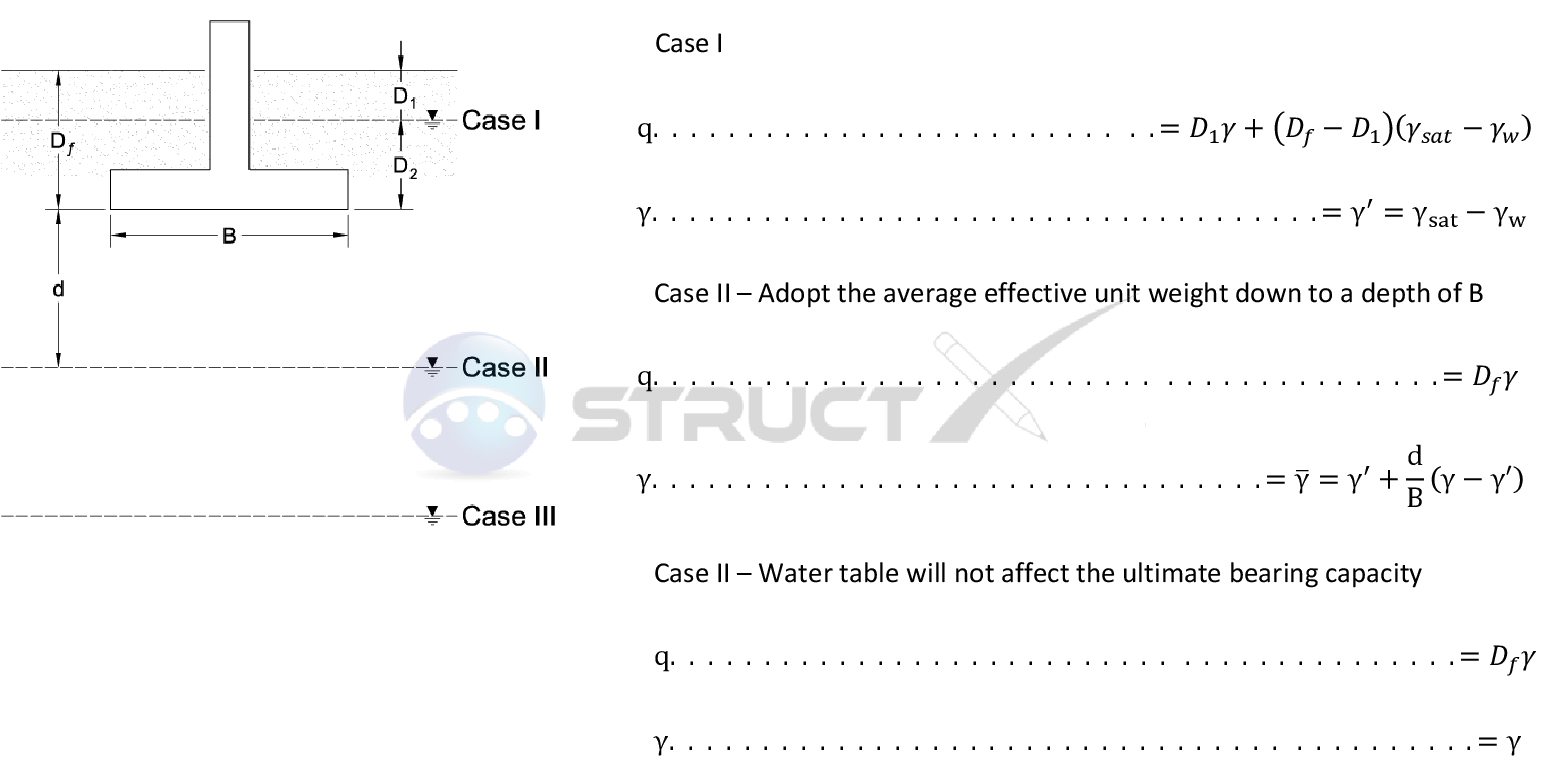# Modification for a Water TableMore Cases

## In the presence of water

In many cases the ultimate bearing capacity has been based on the assumption that the water table is well below the foundation under consideration, to the extent where it will not influence the properties of the soil. Because this is not always the case, modification of bearing capacity equations may be required. Such modifications for dealing with the presence of water have been provided below.# Notation and Units

## Metric and Imperial Units

The above formulas may be used with both imperial and metric units. As with all calculations care must be taken to keep consistent units throughout with examples of units which should be adopted listed below:

# Notation

• B = width of foundation, ft or m
• d = depth of water table below foundation, ft or m
• Df = depth of ground foundation measured from ground surface, ft or m
• D1 = depth of water table from ground surface, ft or m
• D2 = depth of foundation below water table, ft or m
• q = effective unit weight of soil , lb/ft3 or kN/m3
• γ = unit weight of soil, lb/ft3 or kN/m3
• γ' = effective unit weight of soil, lb/ft3 or kN/m3
• γsat = saturated unit weight of soil, lb/ft3 or kN/m3
• γw = unit weight of water, lb/ft3 or kN/m3